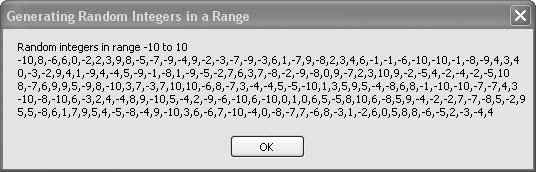# Recipe 6.27. Generating Random Integers in a Range

#### Problem

You need to generate a sequence of pseudorandom integers with a flat distribution over a given range.

#### Solution

Sample code folder: Chapter 06\RepeatRandom

The BetterRandom class (see Recipe 6.26) sports a GetNextInteger() function. Two parameters define the range limits for the returned pseudorandom integer, as shown here:

` newRnd.GetNextInteger(minInt, maxInt) `

The returned integer has a statistically flat distribution across the given range.

#### Discussion

The following code creates a new instance of the BetterRandom object, which it then uses to generate 200 pseudorandom integers in the range -10 to +10. The results are collected and then displayed for review. As a programming exercise, you might consider changing this code to display the average and perhaps the standard deviation for these returned values.

The generator object is created without passing a string to initialize the generator, so a unique sequence is created every time this program is run:

` Dim result As New System.Text.StringBuilder Dim generator As New BetterRandom Dim minInt As Integer = -10 Dim maxInt As Integer = 10 Dim counter As Integer result.Append("Random integers in range ") result.AppendLine(minInt & " to " & maxInt) For counter = 1 To 200    ' ----- Add one random number.    result.Append(generator.GetNextInteger(-10, 10))    If ((counter Mod 40) = 0) Then       ' ----- Group on distinct lines periodically.       result.AppendLine()    Else       result.Append(",")    End If Next counter MsgBox(result.ToString()) `

Figure 6-27 shows the results of generating the 200 pseudorandom integers.

##### Figure 6-27. Pseudorandom integers in the range -10 to +10 generated by the BetterRandom objectRecipe 6.26 shows the full code for the BetterRandom class.

Search Visual Studio Help for "Random Class" and "RNGCryptoServiceProvider Class" for information about other ways to generate pseudorandom numbers in Visual Basic.Visual Basic 2005 Cookbook: Solutions for VB 2005 Programmers (Cookbooks (OReilly))
ISBN: 0596101775
EAN: 2147483647
Year: 2006
Pages: 400

Similar book on Amazon